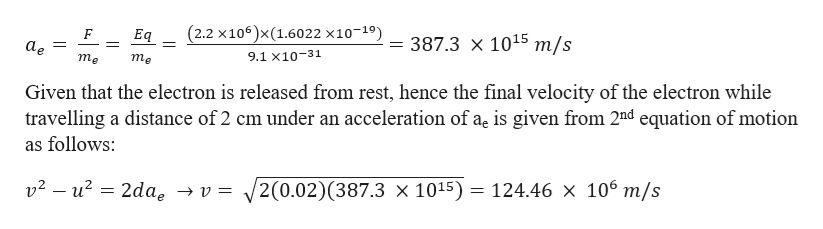# Electric fieldElectron+

Question
1 views

Ch 19, Problem 38

An electron is released from rest at the negative plate of a parallel plate capacitor and accelerates to the positive plate (see the drawing). The plates are separated by a distance of 2.0 cm, and the electric field within the capacitor has a magnitude of 2.2 x 106 V/m. What is the kinetic energy of the electron just as it reaches the positive plate?

check_circle

Step 1

Given information:

Electric field strength between the plates (E) = 2.2 × 106 V/m

Distance between the two plates (d) = 2 cm = 0.02 m

General information:

Mass of the electron (me) = 9.1 x 10-31 kg.

Charge on the electron (qe) = 1.6022 x 10-19 C.

Step 2

The acceleration (ae) of the ele...help_outlineImage Transcriptionclose(2.2 x106)x (1.6022 x10-19) F Eq = 387.3 x 1015 m/s аe = 9.1 х10-31 тe тe Given that the electron is released from rest, hence the final velocity of the electron while travelling a distance of 2 cm under an acceleration of ae is given from 2nd equation of motion as follows v2- u2 2dae -»v = 2(0.02)(387.3 x 1015) = 124.46 x 106 m/s fullscreen

### Want to see the full answer?

See Solution

#### Want to see this answer and more?

Solutions are written by subject experts who are available 24/7. Questions are typically answered within 1 hour.*

See Solution
*Response times may vary by subject and question.
Tagged in

### Physics